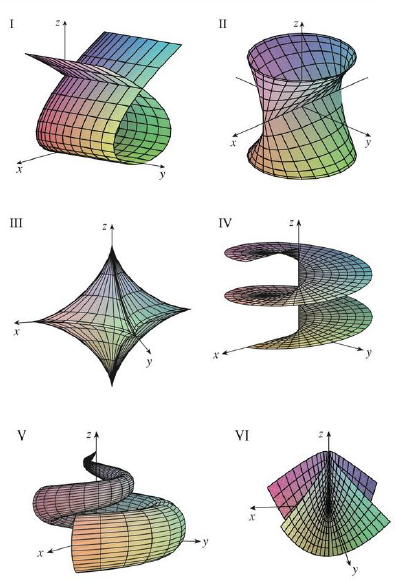Chapter 16.6, Problem 17E

Chapter
Section
Textbook Problem

Match the equations with the graphs labeled I–VI and give reasons for your answers. Determine which families of grid curves have u constant and which have v constant.17. x = cos3u cos3v, y = sin3u cos3v, z = sin3vTo determine

To match: The parametric equations x=cos3ucos3v,y=sin3ucos3v,z=sin3v with the graphs labeled I-VI in the textbook and to explain the reason for the combination.

Explanation

Given data:

Write the given parametric equations as follows.

x=cos3ucos3v,y=sin3ucos3v,z=sin3v

The graphs are labeled in the textbook from I to VI.

Determination of grid curves when v is constant:

If the parameter v is held constant, the parameter z becomes constant and the grid curves lies in a horizontal planes.

Therefore, when the parameter v is constant, the graphs exhibit the horizontal grid curves but the grid curves are neither ellipses nor straight lines

Still sussing out bartleby?

Check out a sample textbook solution.

See a sample solution

The Solution to Your Study Problems

Bartleby provides explanations to thousands of textbook problems written by our experts, many with advanced degrees!

Get Started

g(x)=2(x1)5/2

Applied Calculus for the Managerial, Life, and Social Sciences: A Brief Approach

Evaluate the integrals in Problems 1-26. 10.

Mathematical Applications for the Management, Life, and Social Sciences

True or False: f(x) is symmetric about the origin if f(x) =f(x).

Study Guide for Stewart's Single Variable Calculus: Early Transcendentals, 8th

Find the slope and y-intercept of the line with equation 3x5y=6.

Finite Mathematics for the Managerial, Life, and Social Sciences